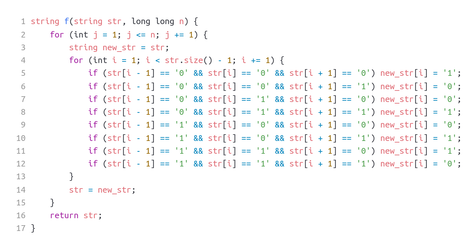# Yet Another Strong ProblemTahseentesting contest11/28/96
Limits 1s, 512 MB

You are given a string $\text{str}$ consisting of characters $0$ and/or $1$ with an arbitrary integer $n$.

Without any reason, I want to evaluate this following function with the value $\text{str}$ and $n$. Unfortunately, my function stops working for larger values. Can you help me to find the actual output of this function?N.B: it is guaranteed initial $\text{str}$ will have zero in the first and last position, and the indices are zero-based on the above function.

## Input

The input is composed of two lines.

The first line of the input contains a string, $\text{str}$$(1\le |\text{str}| \le 100)$ — The first parameter of the function, mentioned in the statement.

The second line contains an integer, $n (0\le n \le 2^{60})$— the second parameter of the function.

## Output

Print the string, output of the function, in a single line.

## Sample

InputOutput
0001011010
10


0010110010


### Submit